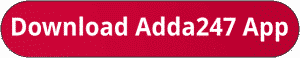Latest Banking jobs   »   IBPS RRB quant

# Quantitative Aptitude Quiz For IBPS RRB PO/Clerk Prelims 2023 -23rd July

Directions (1-5): In each of these questions, two equations (I) and (II) are given. You have to solve both the equations and answer the following questions.
(a) x > y
(b) x < y
(c) x ≥ y
(d) x ≤ y
(e) x = y or no relationDirections (6-10): The table given below shows the no. of books published by 4 different publishers in 4 months. Study the data and answer the following questions.Q6. What is the average number of books published by A in all the given months?
(a) 1740
(b) 2275
(c) 2050
(d) 2175
(e) 2250

Q7. Find the number of books published by B in February and March together is what percent more/less than that by C in March and April? (approximate)
(a) 21%
(b) 24%
(c) 16%
(d) 12%
(e) 27%

Q8. Find the ratio of number of books published by C to D in all given months.
(a) 23 : 45
(b) 24 : 43
(c) 42 : 23
(d) 41 : 25
(e) 23 : 42

Q9. Find the revenue obtained by B in March is how much more/less than that by D in same month, if selling price of book is Rs 120 and all books are sold. (Note – cost price and selling price of each book is same for all publishers)
(a) Rs 50,000
(b) Rs 40,000
(c) Rs 55,000
(d) Rs 70,000
(e) Rs 60,000Solutions## FAQs

### What is the selection process of IBPS RRP PO?

The Selection Process of IBPS RRB PO consists of Prelims, Mains and Interview

#### Congratulations!Union Budget 2023-24: Free PDF